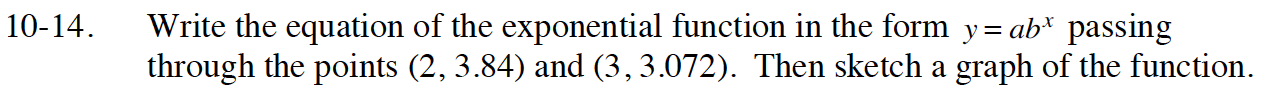### Home > INT1 > Chapter Ch10 > Lesson 10.1.1 > Problem10-14

10-14.

Write the equation of the exponential function in the form y = abx passing through the points (2, 3.84) and (3, 3.072). Then sketch a graph of the function. 10-14 HW eTool (Desmos). Homework Help ✎Homework Help ✎Homework Help ✎Substitute the points into the equation.
3.84 = ab2
3.072 = ab3

Solve 3.84 = ab2 for a, then substitute that into the other equation and solve for b.

Once you have solved for b, substitute that value back into one of the original equations and solve for a.

Check your work using the eTool below. Does your equation pass through the two points plotted on the graph?
Click the link at the right to view full eTool version: Int1 10-14 HW eTool.